Welcome, Guest.

###Stats ezBlockMembers
Total Members: 136
Latest: garee
New This Month: 2
New This Week: 2
New Today: 0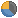Stats
Total Posts: 32933
Total Topics: 1170
Most Online Today: 2580
Most Online Ever: 46271
(March 28, 2021, 08:01:47 pm)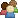Users Online
Members: 3
Guests: 87
Total: 90

###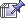Author Topic: Biblical Flat Earth and Cosmos  (Read 32159 times)

0 Members and 0 Guests are viewing this topic.

#### patrick jane

• Sr. Member
•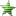•• Posts: 22942
• Karma: +1010/-0
• Research Jesus Christ - Research Flat Earth
• Location: Homeless in God's Flat Earth
• Referrals: 48##### Re: Biblical Flat Earth and Cosmos
« Reply #156 on: June 04, 2021, 03:39:01 am »
Round Earth distance to the sun, and therefore the size of the solar system, relies on the idea that the earth is a sphere. The triangulation method depends on an assumption about the shape of the earth.

Distance to the Sun
Q. Why are the celestial bodies and the sun so close to the earth's surface in the Flat Earth Model?

A. The celestial bodies must be close because if the shape of the earth changes, the distance to the celestial bodies must change as well. Astronomers use two different observations on far off points on earth to triangulate the distance of celestial bodies. When the shape of the earth changes, the triangulation changes, and our perception of the universe must therefore change as well.

Eratosthenes' stick experiment can not only tell us about the size of the earth, but can also be used to compute the distance to the sun as well. If the earth is round, the celestial bodies are computed to be millions of miles distant. If the earth is flat, the celestial bodies are triangulated to be relatively close to the earth's surface.

In his experiment Eratosthenes assumes that the earth is a globe and that the sun is very far away in his computations for the size of the earth and the distance to the sun. However, if we use his data with the assumption that the earth is flat we can come up with a wildly different calculation for the distance of the sun, showing it to be close to the earth. The sun changes its distance depending on the model of the earth we assume for the experiment.

Millersville University goes over the two ways of interpreting Eratosthenes' data. The first part of the article goes over the interpretation of his data under a Round Earth model, and the bottom part of the article goes over an interpretation of the data under a Flat Earth model.

Here's a link which explains the idea: http://www.millersville.edu/physics/experiments/058/index.php. The first part goes over the Round Earth explanation for how the sun can be computed millions of miles distant. At the bottom there is a Flat Earth explanation for how the sun can be computed as being very close to the earth's surface. Scroll all the way to the bottom to the "alternative model" section. You will find that we can use Eratosthenes' data, in conjunction with the assumption of a Flat Earth, to confirm that in FET the sun is very near to the earth's surface.

Hence, if we assume that the earth is flat, triangles and trigonometry can demonstrate that the celestial bodies are fairly close to the earth.

 Eratosthenes' model depends on the assumption that the earth is a globe and that the sun is far away and therefore produces parallel rays of light all over the earth. If the sun is nearby, then shadows will change length even for a flat earth. A flat earth model is sketched below. The vertical stick casts shadows that grow longer as the stick moves to the left, away from the closest point to the sun. (The sun is at height h above the earth.)  A little trigonometry shows that  Using the values 50 degrees and 60 degrees as measured on the trip, with b=1000 miles, we find that h is approximately 2000 miles. This relatively close sun would have been quite plausible to the ancients.

Continuing the calculation, we find that a is approximately 2400 miles and the two distances R1 and R2 are approximately 3000 and 3900 miles, respectively. 

There is no other way to get a distance for the sun. Just looking at it from a single point on earth will not tell you its distance, you must look at it from several points and account for the curvature or non-curvature of the distance between those points.

Please note: The writer of that article makes a unrelated side comment about the Flat Earth model --

 That is, as we move from Florida to Pennsylvania, our distance from the sun increases by about 30%. As a consequence the apparent size of the sun should decrease by 30%. We see no noticeable change in the apparent size of the sun as we make the trip. We conclude that the flat earth/near sun model does not work. 

This has little to do with the distance from the sun via triangulation methods. The writer of the above statement apparently did not read Chapter 10 of Earth Not a Globe. See: Magnification of the Sun at Sunset

Sun's Distance - Zetetic Cosmogony
Thomas Winship, author of Zetetic Cosmogony, provides a calculation demonstrating that the sun can be computed to be relatively close to the earth's surface if one assumes that the earth is flat and that light travels in straight lines --

 On March 21-22 the sun is directly overhead at the equator and appears 45 degrees above the horizon at 45 degrees north and south latitude. As the angle of sun above the earth at the equator is 90 degrees while it is 45 degrees at 45 degrees north or south latitude, it follows that the angle at the sun between the vertical from the horizon and the line from the observers at 45 degrees north and south must also be 45 degrees. The result is two right angled triangles with legs of equal length. The distance between the equator and the points at 45 degrees north or south is approximately 3,000 miles. Ergo, the sun would be an equal distance above the equator. 

Sun's Distance - Modern Mechanics
Modern Mechanics describes how on a Flat Earth the sun can be computed to 3,000 miles via triangulation, whereas on a globe earth those same angles can calculate the sun to nearly 93 million miles away --Get Right

#### patrick jane

• Sr. Member
••• Posts: 22942
• Karma: +1010/-0
• Research Jesus Christ - Research Flat Earth
• Location: Homeless in God's Flat Earth
• Referrals: 48##### Re: Biblical Flat Earth and Cosmos
« Reply #157 on: June 04, 2021, 03:45:34 pm »
AnglesWith RefractionNo RefractionParameter Descriptions

Note: Values marked with a * are not dependent on Refraction in reality. The marked values show the apparent values if refraction is not zero. So to display the real values, set Refraction = 0. This is true for all Horizon Data as well.

Basic Panel
Observer Height: Height of the observer above sea level.

Target Distance: Distance from observer to target along the surface.

Target Size: Height of the target from sea level to the top of the target.

Refraction: Refraction Coefficient k. See Panel Refraction for more parameters. If you click on Std then standard refraction is calculated according to the observer height and standard atmospheric conditions. For show k is calculated see Refraction-Coefficient k.

Zoom, View∠: Zoom factor f = focal length in 35 mm equivalent units or viewing angle can be used to magnify the image. This two parameters are linked by the following equation (see Angle of view):

f = { 43{.}2\ \mathrm{mm} \over 2 \cdot \tan( \theta / 2 ) }

Nearest Target Data Panel

In this panel some calculated object data is displayed. If multiple objects are selected, the data for the nearest object is displayed.

Visible, Hidden: how much of the object size is hidden behind the horizon and how much is visible.

Angular Size, Angular Visible, Angular Hidden: like above but in angular size. The angular size is arctan( size / distance ) in degrees.

Refraction Angle: How much of the object is lifted due to refraction expressed as an angular size. See Refraction-Angle ρ how this angle is calculated.

Lift Absolute: absolute amount of apparent lift of the object with respect to eye level due to refraction.

Relative to Horizon: amount of apparent lift of the object with respect to the horizon due to refraction. The horizon appears lifted with respect to eye level by refraction too. If an object lies behind the horizon, its lift relative to the horizon is smaller than the absolute lift of the object with respect to eye level.

Target Top Angle, Target Top Angle FE: Angle α between target top and eye level for globe and flat earth (FE) respectively. The angle is positive if the target top is above eye level. Some theodolites measure a so called zenith angle ζ. The zenith angle is the angle between the vertical up and the target top. The correlation between this angles is α = 90° − ζ.

Angular Distance θ, Tilt θ: is the angle between the observer, the center of the earth and the nearest target. This angle is used in some Drop calculators, as the Drop x is:

x = R \cdot \big( 1 - \cos( \theta )

where'
'   x ='   'drop from the surface level
'   R ='   'radius of the earth
'   \theta ='   'angle between observer, the center of the earth and the target

Note that  is the same angle as the Tilt of the target object.

Drop: is the amount the surface at the target has dropped from the tangent plane at the surface of the observer. This amount depends on the surface distance between observer and target. This distance is dependent on the Target Distance and the Side Pos of the target via Pythagoras.

Bulge Height: is the maximal amount the surface appears to bulge up from the direct line through the earth from the surface at the observer and the surface at the target. This distance is dependent on the Target Distance and the Side Pos of the target via Pythagoras. Note, because the surface bends down in every direction on the globe, the bulge is always lower than the plane tangential at the surface of observer.

Distance: is the distance along the surface from the base of the observer to the base of the target. It is dependent on the Target Distance and the Side Pos of the target via Pythagoras.

Horizon Data Panel
Dist on Surf: is the distance of the horizon line from the base of the observer along the surface.

Dip Angle: is the angle between the horizon line and the eye level line as measured at the observer.

Dist from Eye: is the line of sight distance of the horizon line from the observer.

Dist on Eye-Lvl: is the distance of the horizon measured on the eye level plane.

Drop from Surf: is the drop of the horizon line as measured down from the tangential plane with origin at the surface of the obsever.

Drop from Eye-Lvl: is the drop of the horizon line as measured down from the tangential plane with origin at the observer height. Drop from Eye−Lvl = Drop from Surf + Height.

Frame Width: Is the width of the frame as measured with a scale at the center of the horizon. This amount depends on the distance of the horizon and the viewing angle or focal length. Compare with Left-Right Width.

Grid Spacing: is the distance between the grid lines of the globe model.

Left-Right Width specifies the horizontal distance between the 2 points where the curved horizon meets the border of the frame. The line between the 2 points passes through the earth. The distance of the line between this 2 points is smaller than the distance to the center of the horizon Dist from Eye.

Left-Right Drop (Angle) are the apparent drop height and angle respectively from the horizon tangent to the line between the 2 points where the curved horizon meets the border of the frame. The drop height is calculated from the drop angle using the distance to the center of the horizon Dist from Eye.

For a derivation of how Left-Right values are calculated see Calculating left-right Horizon Drop.

Radius Earth: is the radius of the earth used for all calculations. This value can be changed.

Exact Equations for the Hidden Height
How much of an object is hidden behind the curvature of the earth, the so called hidden height hh, depends on the distance of the object from the observer and from the height of the observers eye above the surface hO. The distance can be expressed as the line of sight d to the object, tangent to the horizon, or as the arc length s along the surface of the earth between observer and target.

Note: To calculate the hidden height you must not use the famous equation 8 inches per miles squared! This equation is an approximation to calculate the drop of the earth surface from a tangent line on the surface at the observer. It calculates not the hidden part of an object.

Depending on whether you know the line of sight distance d or the distance along the surface s the following equations calculate the hidden height exactly:

h_\mathrm{h} = \sqrt{ { \left[ d - \sqrt{ { ( R + h_\mathrm{O} ) }^2 - { R }^2 } \right] }^2 + { R }^2 } - R

h_\mathrm{h} = { R \over \cos\left[ { s \over R } - \arccos\left( { R \over R + h_\mathrm{O} } \right) \right] } - R

where'
'   h_\mathrm{h} ='   'hidden height
'   d ='   'line of sight distance between observer and target, tangential to the surface of the globe
'   s ='   'distance between observer and target along the surface of the globe
'   h_\mathrm{O} ='   'eye height of the observer measured from the surface of the globe
'   R ='   '6371 km = radius of the earth without refraction; 7433 km = radius to use for standard refraction 7/6 Rearth; 7681 km = radius for standard refraction k = 0.17 (sea level)

The same equations can be used to calculate the hidden height with and without refraction. You simply have to choose the corresponding value for R. Because under standard refraction the earth looks less curved, you can use a bigger radius for the earth than it is in reality. For standard refraction 7/6 · Rearth use R = 7433 km. For standard refraction k = 0.17 use R = 7681 km.

There are multiple slightly different values for standard refraction in use. Near the ground the bigger value is more accurate. For higher altitudes the smaller value is more accurate. If you press the button Std the App calculates refraction depending on the observer altitude. Near sea level refraction is about k = 0.17.

The hidden height equations are only valid if the object lies behind the horizon. That is if the distance to the horizon dH or sH is less than the distance to the target d or s.

The exact distances to the horizon can be calculated with the following equations:

d_\mathrm{H} = \sqrt{ { ( R + h_\mathrm{O} ) }^2 - { R }^2 }

s_\mathrm{H} = R \cdot \arccos\left( { R \over R + h_\mathrm{O} } \right)

where'
'   d_\mathrm{H} ='   'line of sight distance to the horizon
'   s_\mathrm{H} ='   'distance to the horizon along the surface of the globe
'   h_\mathrm{O} ='   'eye height of the observer measured from the surface of the globe
'   R ='   '6371 km = radius of the earth without refraction; 7433 km = radius to use for standard refraction 7/6 Rearth; 7681 km = radius for standard refraction k = 0.17 (sea level)

Approximation Equations for the Hidden Height

If the observer height hO is much smaller than the radius of the earth R, the Exact Equations for the Hidden Height can be simplified by the following approximation:

h_\mathrm{h} \approx { { \left( d - \sqrt{ 2 \cdot R \cdot h_\mathrm{O} } \right) }^2 \over 2 \cdot R }

for   h_\mathrm{O} \ll R

where'
'   h_\mathrm{h} ='   'hidden height
'   h_\mathrm{O} ='   'eye height of the observer measured from the surface of the globe
'   d ='   'distance between observer and target, line of sight or along the surface
'   R ='   '6371 km = radius of the earth without refraction; 7433 km = radius to use for standard refraction 7/6 R earth; 7681 km = radius for standard refraction k = 0.17 (sea level)
Note: for observer height much less than the radius of the earth, the line of sight distance d and the surface distance s are identical for all practical purposes. So the equation above holds for both cases.

The distance to the horizon can also be approximated by the following equation:

d_\mathrm{H} \approx \sqrt{ 2 \cdot R \cdot h_\mathrm{O} }

where'
'   d_\mathrm{H} ='   'distance to the horizon
'   h_\mathrm{O} ='   'eye height of the observer measured from the surface of the globe
'   R ='   '6371 km = radius of the earth without refraction; 7433 km = radius to use for standard refraction 7/6 Rearth; 7681 km = radius for standard refraction k = 0.17 (sea level)

Conclusion: For all practical purposes while the observer is within the troposphere, so that the observer height hO is much less than the radius of the earth R, you can use the approximation equations. In this case for the distance between observer and target you can use the line of sight or the distance along the surface. They are practically identical.

How much the horizon drops at each end of the image depends on the focal length (zoom) of the camera. The curve of the horizon is barely noticable if the viewing angle is narrow (high zoom value). Due to perspective distortions, if we are not thousands of kilometers away from earth, the apparent curve of the horizon is only approximately a circular arc. This gets obvious on very wide angle lenses beyond a viewing angle of 70°. But at the center of each image the apparent radius of the horizon is exactly the refracted radius of the earth (about 7/6 R for standard refraction). The reason is because we are looking tangential to a great circle passing through the horizon point from left to right.

Why does the curvature appear different from different altitudes? Because as we increase the observer altitude the horizon distance gets bigger. Due to perspective, everything at the horizon appears smaller. This includes the radius of the earth at the horizon, which is the apparent radius mentioned here. So although the curvature of the horizon is always the refracted R, it appears different due to perspective and the distance to the horizon.
« Last Edit: June 05, 2021, 07:07:02 am by patrick jane »
Get Right

#### patrick jane

• Sr. Member
••• Posts: 22942
• Karma: +1010/-0
• Research Jesus Christ - Research Flat Earth
• Location: Homeless in God's Flat Earth
• Referrals: 48##### Re: Biblical Flat Earth and Cosmos
« Reply #158 on: June 16, 2021, 12:15:40 pm »
The People of Other Worlds - ROBERT SEPEHR

Dr. Hermann Oberth was a respected Austro-Hungarian-born German physicist and engineer, internationally-known rocket pioneer, and head of the US CALTECH Laboratories until 1955. Considered one of the founding fathers of rocketry and astronautics, Oberth eventually came to work for one of his students, former SS officer Wernher von Braun, who was developing space rockets for NASA.

1 hour
Get Right

#### patrick jane

• Sr. Member
••• Posts: 22942
• Karma: +1010/-0
• Research Jesus Christ - Research Flat Earth
• Location: Homeless in God's Flat Earth
• Referrals: 48##### Re: Biblical Flat Earth and Cosmos
« Reply #159 on: July 01, 2021, 08:29:41 pm »
Uniform Circular Motion is Constant Acceleration

5 minutes

Consult any mainstream science publication or ask any science teacher "why can't we feel the Earth spinning?" and they will unanimously reply that it is because the globe spins at a perfect constant speed, and we can only feel acceleration or deceleration.  As CoolCosmos writes: "Earth moves very fast. It spins at a speed of about 1,000 miles per hour and orbits around the Sun at a speed of about 67,000 miles per hour.

We do not feel any of this motion because these speeds are constant. You can only feel motion if your speed changes. For example, if you are in a car which is moving at a constant speed on a smooth surface, you will not feel much motion. However, when the car accelerates or when the brakes are applied, you do feel motion."  The glaring problem with this claim rarely ever addressed is that the globe and everything on it, if it existed, would be undergoing constant acceleration, because uniform circular motion IS constant acceleration.

As published on physicsclassroom.com, "Uniform circular motion can be described as the motion of an object in a circle at a constant speed. As an object moves in a circle, it is constantly changing its direction. At all instances, the object is moving tangent to the circle. Since the direction of the velocity vector is the same as the direction of the object's motion, the velocity vector is directed tangent to the circle as well. An object moving in a circle is accelerating. An object undergoing uniform circular motion is moving with a constant speed.

Nonetheless, it is accelerating due to its change in direction. The direction of the acceleration is inwards. With the inward net force directed perpendicular to the velocity vector, the object is always changing its direction and undergoing an inward acceleration."  Therefore the globe's theoretical astronomy is at odds with demonstrable physics.  Uniform (and even moreso with non-uniform) circular motion creates a centripetal force which entails constant acceleration in constantly changing directions.

The heliocentric models claims the Earth rotates in uniform circular motion at a constant speed while revolving an elliptical orbit around the Sun at another constant speed, while the entire solar system makes a circular orbit around the Milky Way galaxy at another constant speed, and the Milky Way galaxy shoots off in a straight line from their Big Bang beginning at yet another constant speed.  In other words, we are actually supposedly undergoing 4 different circular, elliptical and straight line motions simultaneously, but being told that we quote "do not feel any of this motion because these speeds are constant."

They always give the example of a car or train going straight at a constant velocity and claim we cannot feel that motion, but what if the car is speeding along an elevated uniform circular racetrack, while that entire elevated racetrack is making an elliptical circuit around the racing grounds, while simultaneously the entire racing grounds themselves are on a gigantic moving circular foundation, while that foundation itself shoots straight off into the universe?  These are the actual variables involved with the globe's supposed constant speed that no one in history has ever demonstrated, measured, felt or experienced whatsoever.

So the next time someone claims you cannot feel the Earth's motion because it spins a constant speed, remind them that uniform circular motion IS acceleration and acceleration IS felt, and when they give you the example of a car driving down a straight road, remind them that the car is actually driving on a circular racetrack, while the racetrack is making an elliptical circuit around the racing grounds, while the entire racing grounds themselves are circling around a larger foundation, and that foundation itself is traveling in a straight line.

These 4 simultaneous varying motions the globe is subjected to in the heliocentric model create multiple acceleration vectors and destroy any possibility that they wouldn't be felt or measurable due to traveling at a constant speed.
Get Right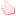Like x 1 View List

#### patrick jane

• Sr. Member
••• Posts: 22942
• Karma: +1010/-0
• Research Jesus Christ - Research Flat Earth
• Location: Homeless in God's Flat Earth
• Referrals: 48##### Re: Biblical Flat Earth and Cosmos
« Reply #160 on: July 17, 2021, 05:34:37 pm »
Space Virgins Get Lucky?

3 minutes

Space fantasy fan-boys and fan-girls have just received their latest damage-control propaganda update from actor Richard Branson where he claims to have gone to space and filmed the spinning-ball Earth for us (but really he just did some parabolic maneuvers simulating free-fall while in a fancy plane)!

Unfortunately for old Dick and his riders, rather than use a normal lens to film the Earth, like all honest amateurs do when sending up their high altitude balloons, these space virgins, just like NASA, Space X, the Red Bull dive and all other so-called "official" sources, always suspiciously choose to use a fish-eye lens causing the horizon to constantly and radically warp from convex, to flat, to concave.

As the camera tilts relative to the horizon the ends warp up or down respectively, but when held still and level the horizon is flat.  As the normal back camera shows, the horizon actually remains perfectly flat and rises to the eye-level of the camera all the way up.

If the Earth was actually a globe, no matter how large, the surface of the globe would curve downwards in all directions away from the observer causing them to necessarily tilt their heads down more and more the further they ascended to be able to see the horizon.  In reality, which is only consistent with rising over a flat plane, the horizon rises along with the observer and remains precisely at eye-level for the entire ascent!

This trick is apparent both in Branson's trip this week as well as Baumgartner's Red Bull dive from several years ago.  The fish-eye lens cameras show a constantly warping horizon which was already curving the picture at ground level the very same amount of so-called "Earth curve" that it showed at 128,000 feet just before diving.

The trick is given away however when they switch to the regular non-fish eye lens camera inside the craft which shows a perfectly flat horizon still perfectly at eye-level while hovering over 20 miles above a supposed globe.  Do you see something wrong with this picture?  If you do, then welcome to the truth of your hoaxed reality.  If you don't, then enjoy watching these terrible slack-jawed actors with their mouths gaping wide open at how ridiculously gullible you sheeple are.

« Last Edit: July 20, 2021, 03:39:11 pm by patrick jane »
Get Right

#### patrick jane

• Sr. Member
••• Posts: 22942
• Karma: +1010/-0
• Research Jesus Christ - Research Flat Earth
• Location: Homeless in God's Flat Earth
• Referrals: 48##### Re: Biblical Flat Earth and Cosmos
« Reply #161 on: July 22, 2021, 05:52:25 am »
BECOME A FLAT EARTHER IN 13 MINUTES

13 minutes
Only available on my BitChute channel
https://www.bitchute.com/video/iaMV4bzmJdw/
Get Right

### Related Topics

Subject / Started by Replies Last post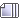91 Replies
12729 Views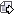July 17, 2021, 05:35:16 pm
by patrick jane28 Replies
10799 ViewsMay 01, 2021, 07:42:33 am
by patrick jane42 Replies
12778 ViewsMarch 14, 2021, 07:10:12 pm9 RepliesSeptember 13, 2020, 12:00:34 pm9 RepliesMay 06, 2021, 08:14:55 pm